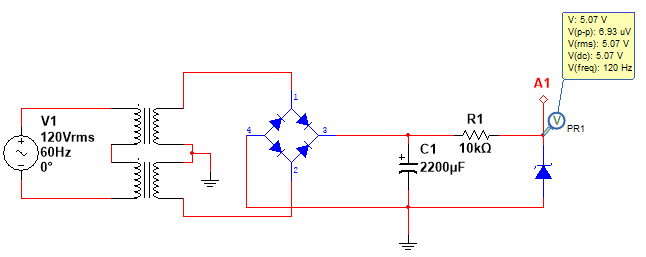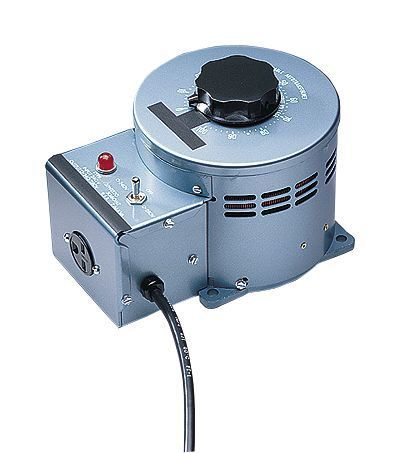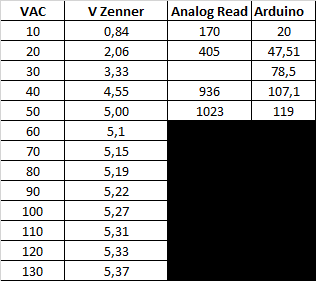# Help to create a VAC sensor

Hi,

I've review many pages from this forum and I couldn't find enough information to use on my project.

I need to create a VAC sensor and read the value through an analog input on arduino. I've created a circuit (5V power supply) to reduce the voltage, by using a transformer 120V:12V, bridge diodes, capacitors and a 5.1V zener diode, since maximun voltage reading from analog inputs on Arduino is 5V.Here is the code that I've created

``````const int sensPin= A2;
int sensorVAC = 0;
float outVAC = 0;
float realValue=0;
int lecturaPin =0;

void setup() {
// put your setup code here, to run once:

Serial.begin (9600);
pinMode(sensPin, INPUT);

}

float sensVAC(){

realValue= (outVAC*24); // (120V/12V equal to 10V), (12V/5V equal to 2,4) (10V*2,4 = 24V)
return realValue;
}

void loop() {
// put your main code here, to run repeatedly:

Serial.print("VAC measurement:     "); //Print the real voltage value after conversion
Serial.println(sensVAC());
delay(1);
}
``````

I used a variable voltage controller to adjust the Vin voltaje on the power supply in order to see the changes on the output voltage on the power supply and the Arduino. The thing is that I am not getting the same voltage value compared with reference voltage.I performed some measurements between the VAC input and Vout on the power supply and it seems that at 50VAC the zener diode reaches the maximum value and also the Arduino reads 1023, so the result at the serial monitor is not as expected.Do you know, how can I get a real measurement on the serial monitor?

With a big storage capacitor, you are simply charging it and then reading the value. There is no discharge path of any consequence.

When the voltage across the bridge gets to about 5Vac, the C will charge to 5v or so and the output will be the voltage across the zener.

Weedpharma

A few things: Firstly, such a circuit can only be indicative of the AC voltage you are wanting to measure, it will not be highly accurate.

But, to improve on it as the circuit is obviously peaking out at a VAC of just under 50Vac, you need to reduce the voltage, either by using a transformer with a lower secondary or by using a resistive voltage divider network.

I would look to having a resistive divider network that allows for 10% higher than your normal expected primary side VAC. Use this voltage to then work out your resistive network so that it is under the Vcc of your Arduino analog input reference, typically 5 Vdc.

The large capacitor you have after the bridge is too large for such a circuit, as there is effectively no load, and will only slow down the response rate. A value of 100µF would suffice fine.

I would place a small 0.1µF cap across the zener diode to shunt any noise at that point from getting into the analog input.

If you want any sort of precision and depending of the range of primary side you wish to measure, then an entirely different circuit will be needed, maybe even in terms of ADC.

I am curious why are there commas after the numbers in your table, if it is decimal notation, then the use of a decimal point is what is used ?

Also, you will need to apply a factor in your calculation to obtain results that reflect a value closer to the true value.

Ah, weedpharma, you beat me to it I see :)

Paul

I believe your x24 here realValue= (outVAC*24); is throwing things off. Your (analogRead values x .00488) are approximately the analog value coming in if Aref = 5V. If Aref is not 5V, the calculated values will be off.

Also, the rectified voltage needs a square root of 2 in there somewhere to determine the DC level from the incoming AC level.

With a center tapped output transformer, pins 3 & 4 are normally used for + and - voltages, see "Full Wave Rectifier" here http://www.play-hookey.com/ac_theory/power_supply/ps_rectifiers.html Your connections have the negative supply grounded, I would remove the center tap Gnd connection so you have just a "Full Wave Bridge Rectifier" for a single positive output.

El_Herrero: